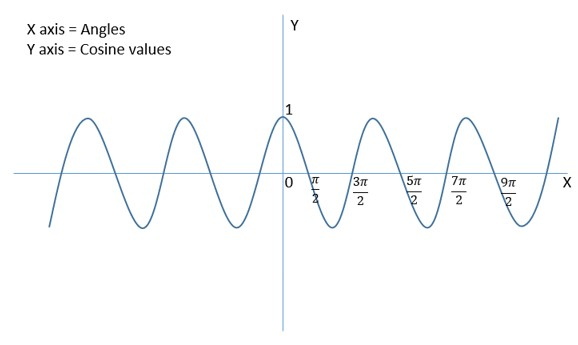# SQL - COS() Function

The SQL COS() function calculates the trigonometric cosine of the given value. This function accepts a single numeric value as an argument. The domain of the argument must be (-∞, ∞) i.e. the set of all real numbers and the range of the result will be [-1,1]. If the value passed to this function doesn't lie in the given domain, it raises an error.

The cosine of an angle is defined as the ratio of the adjacent side of the said angle to the hypotenuse in a right-angled triangle. In mathematics, the most commonly used cosine values are of angles 0, 30, 45, 60 and 90 degrees. The cosine graph for these angles can be seen as below −### Syntax

Following is the syntax of SQL COS() function −

```COS(number)
```

where, number is the value for which we need to calculate the cosine, in radians. The domain of the value is all real numbers.

### Example

If we pass a positive value as an argument, then this function returns it's equivalent cosine value as shown below −

```SELECT COS(8)
AS cosine_value
```

When we run above program, it produces following result −

```+--------------------+
| cosine_Value       |
+--------------------+
| -0.145500033808614 |
+--------------------+
```

### Example

In here, we are trying to pass a negative value as an argument to this function as shown below −

```SELECT COS(-76.32435)
AS cosine_value
```

While executing the above code we get the following output −

```+-------------------+
| cosine_Value      |
+-------------------+
| 0.600934713211343 |
+-------------------+
```

### Example

We can also pass the mathematical constant PI as an argument to this function, it returns it's equivalent cosine value.

```SELECT COS(PI())
AS cosine_value
```

Following is an output of the above code −

```+-------------------+
| cosine_Value      |
+-------------------+
| -1                |
+-------------------+
```

### Example

The cosine value of 0 is 1.

```SELECT COS(0)
AS cosine_value
```

Output of the above code is as follows −

```+--------------+
| cosine_Value |
+--------------+
| 1            |
+--------------+
```

### Example

Assume we have created a table with name CUSTOMERS as shown below −

```create table CUSTOMERS(ID INT NOT NULL,
NAME VARCHAR(20) NOT NULL,
AGE INT NOT NULL,
SALARY DECIMAL(18, 2),
PRIMARY KEY(ID));
Commands completed successfully.
```

Let us insert r values into it −

```insert INTO CUSTOMERS VALUES(1, 'Ramesh', 32, 'Ahmedabad', 2000.00);
insert INTO CUSTOMERS VALUES(2, 'Khilan', 25, 'Delhi', 1500.00);
insert INTO CUSTOMERS VALUES(3, 'kaushik', 23, 'Kota', 2000.00);
insert INTO CUSTOMERS VALUES(4, 'Chaitali', 25, 'Mumbai', 6500.00);
insert INTO CUSTOMERS VALUES(5, 'Hardik', 27, 'Bhopal', 8500.00);
insert INTO CUSTOMERS VALUES(6, 'Komal', 22, 'MP', 4500.00);
insert INTO CUSTOMERS VALUES(7, 'Muffy', 24, 'Indore', 10000.00);
```

Following query calculates the cosine value of the salary of all the customers −

```SELECT NAME, AGE, SALARY,
COS(SALARY)
AS cosine_salaryvalue
FROM CUSTOMERS
```

The result produced is as follows −

```+----------+-----+----------+--------------------+
| NAME     | AGE | SALARY   | cosine_salaryvalue |
+----------+-----+----------+--------------------+
| Ramesh   |  32 |  2000.00 | -0.367459549100831 |
| Khilan   |  25 |  1500.00 | -0.110267402513729 |
| kaushik  |  23 |  2000.00 | -0.367459549100831 |
| Chaitali |  25 |  6500.00 | -0.998996660248886 |
| Hardik   |  27 |  8500.00 |  0.40874243842229  |
| Komal    |  22 |  4500.00 |  0.325439286234295 |
| Muffy    |  24 | 10000.00 | -0.952155368259015 |
+----------+-----+----------+--------------------+
```
sql-numeric-functions.htm